GFG App
Open AppBrowser
Continue

# How to Fix Excel Formulas that are Not Working properly?

Microsoft Excel is a spreadsheet application that has been used by millions of people worldwide for more than three decades. Pivot tables, graphing tools, calculations, and a macro programming language are included. In contrast to other apps such as Word, spreadsheet software calculates values using mathematical formulas and data in cells. However, there are times when the Excel formulas do not work properly. This article will assist you in fixing Excel formula issues.

## How to Fix Excel formulas not working

If an Excel formula is not working properly and returns an error or incorrect result. To fix it, follow the steps below:

1. Change the calculation options from Manual to Automatic.

If you are unable to update the value you have input and it returns the same value, Excel’s calculation option may be set to manual rather than automatic.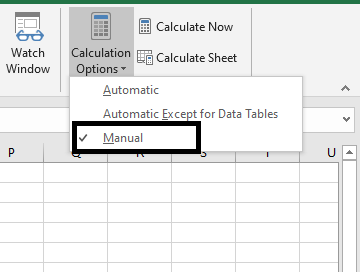Change the calculation option from Manual to Automatic to fix this issue:

• Open the spreadsheet that is giving you problems Go to the Formulas tab, then Select the Calculation option.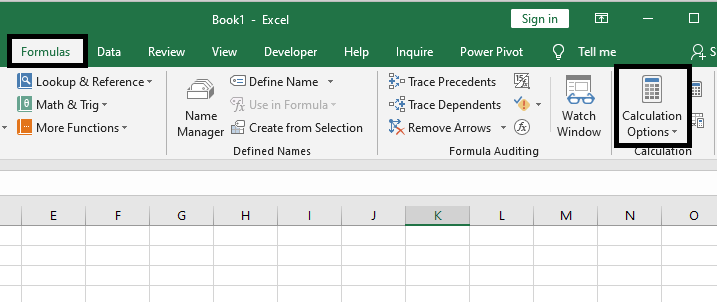• From the dropdown option, select Automatic.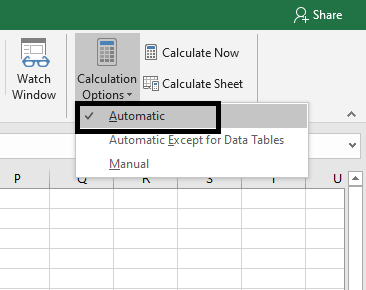2. Change the Cell from Text Format to either General or Number.

You may have formatted the cells containing the formulas incorrectly as text. When Excel is set to text format, it ignores the applied formula and does not display the result.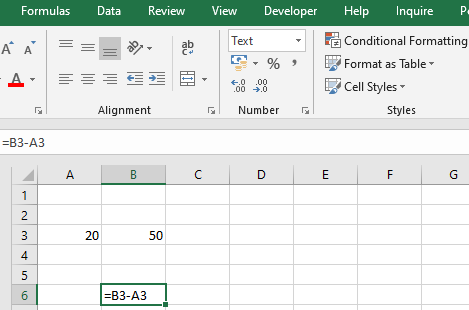To resolve this issue, follow the procedures below:

Step 1: Select the cell where the formula is not being calculated.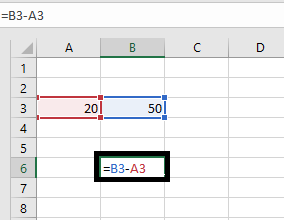Step 2: Navigate to Home, and you’ll see a dropbox with multiple data kinds in the center. Choose General or Number.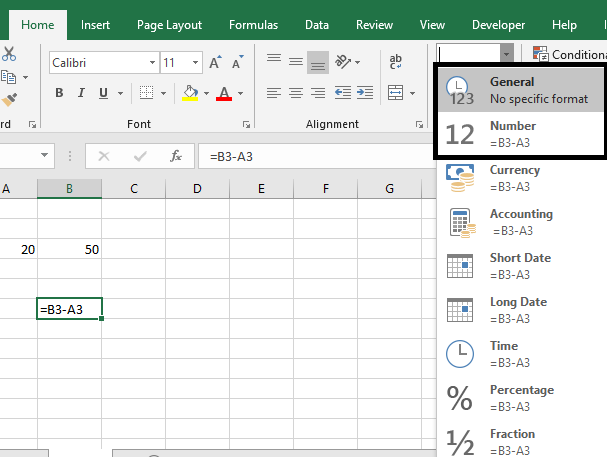Step 3: Now, click the cell once more and Select Enter. The formula will be automatically calculated, and the result will be displayed in the cell.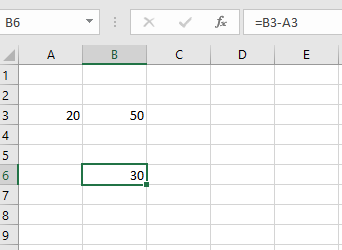3. Turn off the Show Formulas Button.

The Show Formula button is intended for auditing formulas, therefore when selected, it displays the formula rather than the result. As a result, if you previously turned it on, turning it off may help remedy the problem. Here’s how to go about it:

• Navigate to the Formula Tab and click the Show Formulas button under the Formula Auditing Group.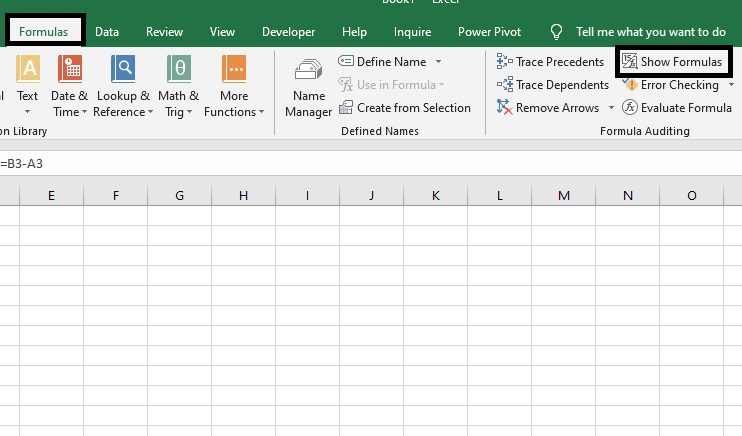## Excel formulas Not Updating

The value provided by your Excel formula does not automatically update, which means that the formula displays the old value even after you modify the values of the dependent cells. When Excel formulas do not update automatically, it is most likely because the Calculation setting has been set to Manual rather than Automatic. To resolve this, simply return the Calculation option to Automatic:

• Navigate to the Formulas tab on the Excel ribbon, then to the Calculation group, click the Calculation Options button, and then select Automatic.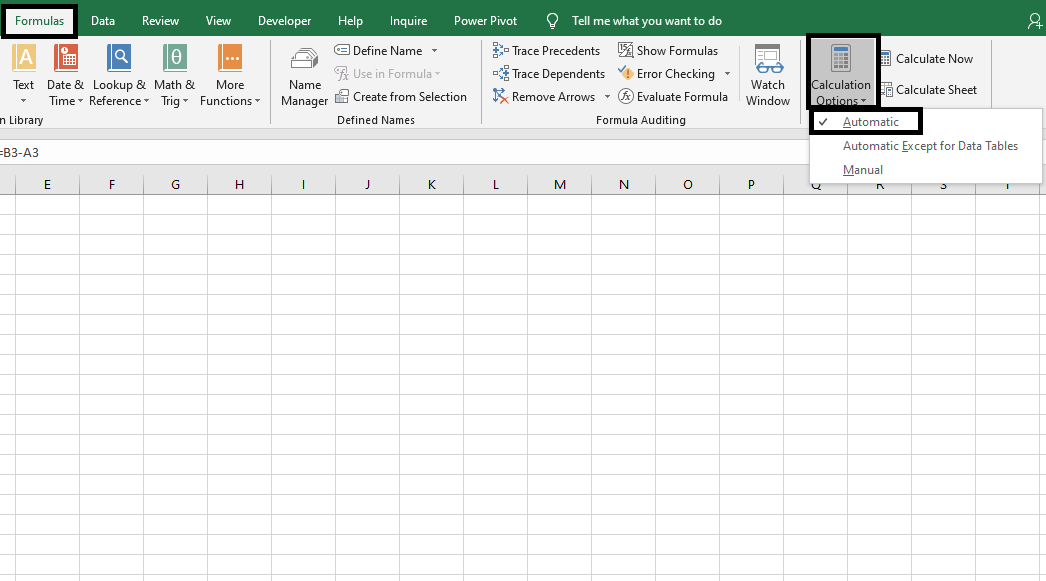## Excel formulas Not Calculating

If your Excel formula isn’t working because a cell displays the function rather than the calculated value, it could be due to one of the following issues.

### Show Formulas Mode is Turned On

The Show Formula button is intended for auditing formulas, therefore when selected, it displays the formula rather than the result. As a result, if you previously turned it on, turning it off may help remedy the problem. Here’s how to go about it.

• Navigate to the Formula Tab and click the Show Formulas button under the Formula Auditing Group.### Formula is Entered as Text

You may have formatted the cells containing the formulas incorrectly as text. When Excel is set to text format, it ignores the applied formula and does not display the result.To resolve this issue, follow the procedures below:

Step 1: Select the cell where the formula is not being calculated.Step 2: Navigate to Home, and you’ll see a dropbox with multiple data kinds in the center. Choose General or Number:Step 3: Now, click the cell once more and Select Enter. The formula will be automatically calculated, and the result will be displayed in the cell.My Personal Notes arrow_drop_up